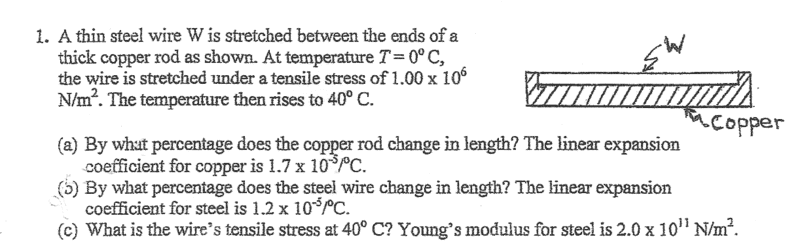# Thermal Expansion of a Wire Connected To a Rod

## Homework StatementΔL=LαΔT
σ=EΔL/L

## The Attempt at a Solution

For part a, I used the coefficient of linear expansion for copper and the change in temperature to find the change in length (0.068%). I thought part b had the same answer. The two are attached, and I would imagine that the elongation would be controlled by the thick rod rather than the thin wire. For part c , I found the length the natural strain of the wire due to thermal expansion (0.048%). I then took the difference between the actual strain and the natural strain and multiplied by young's modulus to find the additional stress (2.4*10^7 N/m^2). I then added that to the original stress (1.00*10^6 N/m^2) to get 2.5*10^7 N/m^2. Is this the correct approach? Thanks.

#### Attachments

haruspex
Homework Helper
Gold Member
2020 Award
For part c , I found the length the natural strain of the wire due to thermal expansion (0.048%).
Fine to here, but I am not quite clear on what you did next. Please post the details.

I knew that the wire would stretch 0.048% without the rod, but it is actually stretched 0.068%. I took the difference of the two values to find the additional strain due to the rod stretching the wire. I then multiplied that by Young's Modulus to find the additional stress:

σ=(2.0*10^11 N/m^2)(0.068%-0.048%)=2.4*10^7 N/m^2.

I then added that stress to the original stress the wire was under (1.00*10^6 N/m^2). This gave me the answer 2.5*10^7 N/m^2.

Last edited:
haruspex
Homework Helper
Gold Member
2020 Award
σ=(2.0*10^100 N/m^2)(0.068%-0.048%)=2.4*10^7 N/m^2.
I guess you meant 1011, not 10100, but the 2.4 is wrong.

Oh, I realized I made a careless error. It is 2*10^-4 . The final answer becomes 41*10^6 N/m^2. The answers just came up, and it's correct.

Last edited:
haruspex
Homework Helper
Gold Member
2020 Award
Yes, I meant 10^11
But the 2.4 is still wrong.

Chestermiller
Mentor

## Homework Statement

View attachment 235607

ΔL=LαΔT
σ=EΔL/L

## The Attempt at a Solution

For part a, I used the coefficient of linear expansion for copper and the change in temperature to find the change in length (0.068%). I thought part b had the same answer. The two are attached, and I would imagine that the elongation would be controlled by the thick rod rather than the thin wire. For part c , I found the length the natural strain of the wire due to thermal expansion (0.048%). I then took the difference between the actual strain and the natural strain and multiplied by young's modulus to find the additional stress (2.4*10^7 N/m^2). I then added that to the original stress (1.00*10^6 N/m^2) to get 2.5*10^7 N/m^2. Is this the correct approach? Thanks.
I think that your conceptual approach is correct. I'm not so sure that you did the arithmetic correctly.